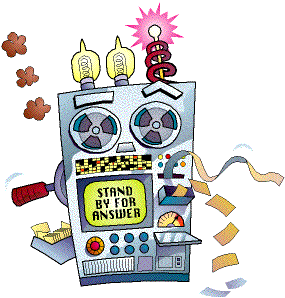# The Amazing Decision Maker

The Decision Maker will help you make a decision by organizing what you already know. You'll need to have a little bit of info about your decision like, what are your options, what considerations influence your decision, and how important are each of these considerations.

The Decision Maker will lead you through five steps:

1. The Decision - What are you trying to decide?
2. The Choices - What are the chioces you have before you?
3. The Factors - What factors influence your choice?
4. Rate the Factors - How important are each of the factors?
5. Rate the Choices -How does each choice rate with regard to each factor?

Each step will guide you through the process, and you can return to any step.

What kind of decision you are trying to make?

In the next step, we will look at the choices you are considering.

# The Choices

Here you are going to identify what choices you have available. This step is easy and you probably already knew what you were trying to choose before you got here.

In the next step, we will look at the factors that influence your decision.

# The Factors

This may require some careful thought. If your factors overlap, you may unintentionally bias your decision. So keep them independent and non-overlapping. You also want to have as few factors as necesssary. Ultimately, you'll have to evaluate how each choice rates with regard to each factor (so 5 choices with 10 factors will result in 50 ratings!).

You'll get better decisions, if you...

• Independent - Make sure each factor is independent of the others.
• Positive - Keep them positive, so that a high score on a particular factor means it is more desireable.
• Minimal - Keep the number of factors as small as possible.

In the next step, we will rate how important each of these factors is relative to the others.

# Rate The Factors

Here you will tell how generally important each factor is by giving it a rating from 1 to 10 (with 10 being the highest). In your results, each of these factors will be weighed according to this rating.

It may be easiest to pick the most important factor and start by giving it a 10. After that, scale your other factors down from that.

Considering just the factors that influence your decision about , how important are each of those factors?

# Rate The Choices

Here you will tell how each of your choices rate considering only one factor at a time. That's worth repeating: Considering only one factor at a time, you will rate each choice on how well it satisfied that one factor.

You will give each choice a rating on how well it satisfied the given criteria on a 1 to 10 scale (with 10 being the highest).